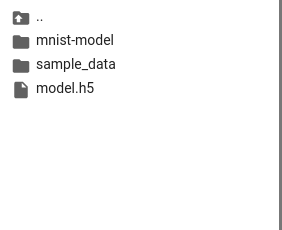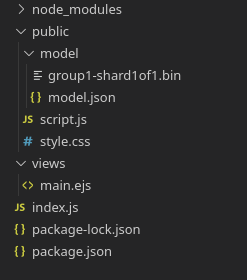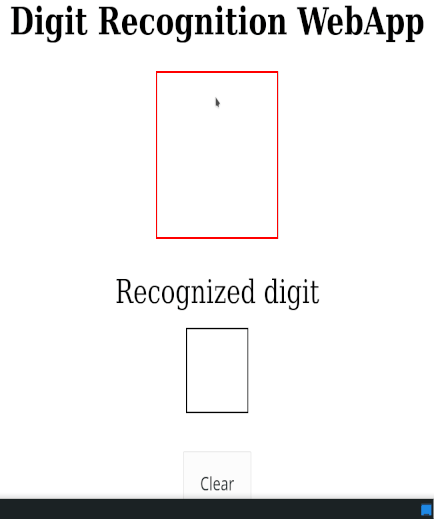# How to deploy a machine learning model using Node.js ?

• Last Updated : 26 Jul, 2021

In this article, we will learn how to deploy a machine learning model using NodeJS. While doing so we will make a simple handwritten digit recognizer using NodeJS and tensorflow.js.

Tensorflow.js is an ML library for JavaScript. It helps to deploy machine learning models directly into node.js or a web browser.

Hey geek! The constant emerging technologies in the world of web development always keeps the excitement for this subject through the roof. But before you tackle the big projects, we suggest you start by learning the basics. Kickstart your web development journey by learning JS concepts with our JavaScript Course. Now at it's lowest price ever!

Training the Model: For training the model we are going to use Google Colab. It is a platform where we can run all our python code, and it comes loaded with most of the machine learning libraries that are used.

Below is the code for the final model which we will create.

## Python

 `# Importing Libraries``from` `tensorflow.keras.datasets ``import` `mnist``import` `matplotlib.pyplot as plt``from` `keras.utils ``import` `np_utils``from` `keras ``import` `Sequential``from` `keras.layers ``import` `Dense``import` `tensorflowjs as tfjs`` ` `# Loading data``(X_train, y_train), (X_test, y_test) ``=` `mnist.load_data()``print` `(``"X_train.shape: {}"``.``format``(X_train.shape))``print` `(``"y_train.shape: {}"``.``format``(y_train.shape))``print` `(``"X_test.shape: {}"``.``format``(X_test.shape))``print` `(``"y_test.shape: {}"``.``format``(y_test.shape))`` ` `# Visualizing Data``plt.subplot(``161``)``plt.imshow(X_train[``3``], cmap``=``plt.get_cmap(``'gray'``))``plt.subplot(``162``)``plt.imshow(X_train[``5``], cmap``=``plt.get_cmap(``'gray'``))``plt.subplot(``163``)``plt.imshow(X_train[``7``], cmap``=``plt.get_cmap(``'gray'``))``plt.subplot(``164``)``plt.imshow(X_train[``2``], cmap``=``plt.get_cmap(``'gray'``))``plt.subplot(``165``)``plt.imshow(X_train[``0``], cmap``=``plt.get_cmap(``'gray'``))``plt.subplot(``166``)``plt.imshow(X_train[``13``], cmap``=``plt.get_cmap(``'gray'``))`` ` `plt.show()`` ` `# Normalize Inputs from 0–255 to 0–1``X_train ``=` `X_train ``/` `255``X_test ``=` `X_test ``/` `255``# One-Hot Encode outputs``y_train ``=` `np_utils.to_categorical(y_train)``y_test ``=` `np_utils.to_categorical(y_test)``num_classes ``=` `10`` ` `# Training model``x_train_simple ``=` `X_train.reshape(``60000``, ``28` `*` `28``).astype(``'float32'``)``x_test_simple ``=` `X_test.reshape(``10000``, ``28` `*` `28``).astype(``'float32'``)``model ``=` `Sequential()``model.add(Dense(``28` `*` `28``, input_dim``=``28` `*` `28``, activation``=``'relu'``))``model.add(Dense(num_classes, activation``=``'softmax'``))``model.``compile``(loss``=``'categorical_crossentropy'``, ``        ``optimizer``=``'adam'``, metrics``=``[``'accuracy'``])``model.fit(x_train_simple, y_train, ``        ``validation_data``=``(x_test_simple, y_test))`

Step 1: Training Data

For training the model, we will be using the MNIST database. This is a large database of handwritten digits which is available for free. In this data set, there are 60,000 images, all of them are of size 28 x 28 pixels in grayscale, with pixel values from 0 to 255.

Step 2: Data Pre-processing

We do the following steps to process our data:

1. Normalize inputs: The inputs are in the range 0-255. We need to scale them to 0-1.
2. One hot encode the outputs

Step 3: Machine Learning

For training the model we use a simple neural network with one hidden layer which is good enough to give about 98% accuracy.

Step 4: Converting the model using tensorflow.js

First, save the model using the following command:

`model.save(“model.h5”)`

Then install tensorflow.js and convert the model using the following command:

```!pip install tensorflowjs
!tensorflowjs_converter --input_format keras
‘/content/model.h5’ ‘/content/mnist-model’```

After running the above command, now refresh the files. Your content should look like the following.Creating Express App and Installing Module:

Step 1: Create package.json using the following command:

`npm init`

Step 2: Now install the dependencies by following the command. We will be using express for server and ejs as template engines.

`npm install express ejs`

Project Structure: Now make sure you have the following file structure. Copy the files that we downloaded from Colab in the model folder.Now write down the following code to your index.js file.

## index.js

 `// Requiring module``const express = require(``"express"``);``const app = express();``const path = require(``"path"``)`` ` `// Set public as static directory``app.use(express.static(``'public'``));`` ` `app.set(``'views'``, path.join(__dirname, ``'/views'``))`` ` `// Use ejs as template engine``app.set(``'view engine'``, ``'ejs'``);``app.use(express.json());``app.use(express.urlencoded({ extended: ``false` `}));`` ` `// Render main template``app.get(``'/'``,(req,res)=>{``    ``res.render(``'main'``)``})`` ` `// Server setup``app.listen(3000, () => {``  ``console.log(``"The server started running on port 3000"``) ``});`

## main.ejs

 ```<``html` `lang``=``"en"``>`` ` `<``head``>``    ``<``script` `src``=``"https://code.jquery.com/jquery-2.2.4.min.js"``>``    ````    ``<``script` `src``=``"https://cdn.jsdelivr.net/npm/@tensorflow/tfjs@1.5.2/dist/tf.min.js"``>``    ````    ``<``link` `rel``=``"stylesheet"` `href``=``"style.css"` `/>```` ` `<``body``>``    ``<``h1``>Digit Recognition WebApp`` ` `    ``<``div` `id``=``"paint"``>``        ``<``canvas` `id``=``"myCanvas"``>``    ```` ` `    ``<``div` `id``=``"predicted"``>``        ``Recognized digit``        ``<``div` `id``=``"number"``>``        ``<``button` `id``=``"clear"``>Clear``    ````     ` `    ``<``script` `src``=``"script.js"``>```` ` ``

## style.css

 `body {``    ``touch-action: ``none``; ``    ``font-family``: ``"Roboto"``;``}``h``1` `{``    ``margin``: ``50px``;``    ``font-size``: ``70px``;``    ``text-align``: ``center``;``}``#paint {``    ``border``:``3px` `solid` `red``;``    ``margin``: ``auto``;``}``#predicted { ``    ``font-size``: ``60px``;``    ``margin-top``: ``60px``;``    ``text-align``: ``center``;``}``#number {``    ``border``: ``3px` `solid` `black``;``    ``margin``: ``auto``;``    ``margin-top``: ``30px``;``    ``text-align``: ``center``;``    ``vertical-align``: ``middle``;``}``#clear {``    ``margin``: ``auto``;``    ``margin-top``: ``70px``;``    ``padding``: ``30px``;``    ``text-align``: ``center``;``}`

Writing the Script: Using the HTML5 canvas we define the mouse events. Then we capture the image on the mouse up and scale it to 28×28 pixels, so it matches our model, and then we pass it to our predict function.

## script.js

 `canvas.addEventListener(``'mousedown'``, ``function` `(e) {``    ``context.moveTo(mouse.x, mouse.y);``    ``context.beginPath();``    ``canvas.addEventListener(``'mousemove'``, onPaint, ``false``);``}, ``false``); ``var` `onPaint = ``function` `() {``    ``context.lineTo(mouse.x, mouse.y);``    ``context.stroke();``};`` ` `canvas.addEventListener(``'mouseup'``, ``function` `() {``    ``\$(``'#number'``).html(``''``);``    ``canvas.removeEventListener(``'mousemove'``, onPaint, ``false``);``    ``var` `img = ``new` `Image();``    ``img.onload = ``function` `() {``        ``context.drawImage(img, 0, 0, 28, 28);``        ``data = context.getImageData(0, 0, 28, 28).data;``        ``var` `input = [];``        ``for` `(``var` `i = 0; i < data.length; i += 4) {``            ``input.push(data[i + 2] / 255);``        ``}``        ``predict(input);``    ``};``    ``img.src = canvas.toDataURL(``'image/png'``);``}, ``false``);`` ` `// Setting up tfjs with the model we downloaded``tf.loadLayersModel(``'model / model.json'``)``    ``.then(``function` `(model) {``        ``window.model = model;``    ``});`` ` `// Predict function``var` `predict = ``function` `(input) {``    ``if` `(window.model) {``        ``window.model.predict([tf.tensor(input)``            ``.reshape([1, 28, 28, 1])])``            ``.array().then(``function` `(scores) {``                ``scores = scores;``                ``predicted = scores``                    ``.indexOf(Math.max(...scores));``                ``\$(``'#number'``).html(predicted);``            ``});``    ``} ``else` `{`` ` `        ``// The model takes a bit to load, ``        ``// if we are too fast, wait``        ``setTimeout(``function` `() { predict(input) }, 50);``    ``}``}`

Step to run the application: Run the index.js file using the following command.

`node index.js`

Output: Open the browser and go to http://localhost:3000, we will see the following output.My Personal Notes arrow_drop_up